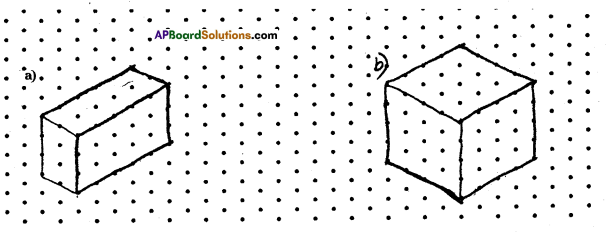AP State Syllabus AP Board 7th Class Maths Solutions Chapter 14 Understanding 3D and 2D Shapes Ex 3 Textbook Questions and Answers.

## AP State Syllabus 7th Class Maths Solutions 14th Lesson Understanding 3D and 2D Shapes Exercise 3Question 1.
Use an isometric dot paper and make an isometric sketch for each one of the given shapes.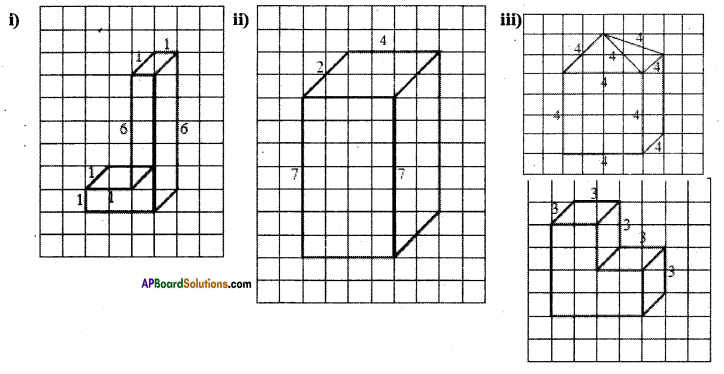Solution: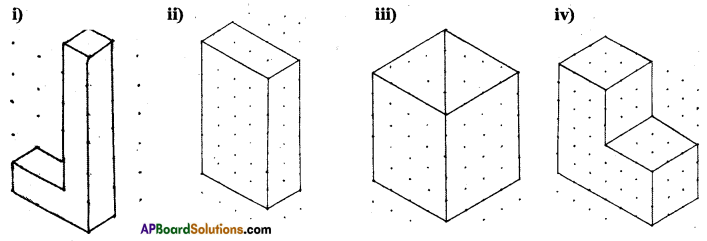Question 2.
The dimensions of a cuboid are 5 cm, 3 cm and 2 cm. Draw three different isometric sketches of this cuboid.
Solution: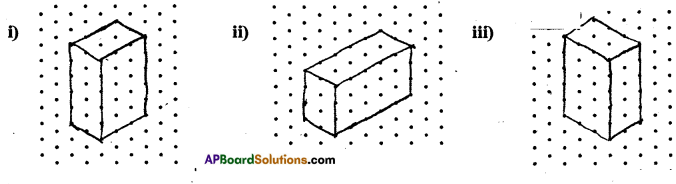Question 3.
Three cubes each with 2 cm edge are placed side by side to form a cuboid. Draw an oblique or isometric sketch of this cuboid.
Solution: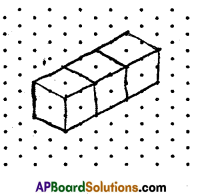Question 4.
Make an oblique sketch for each of the given isometric shapes.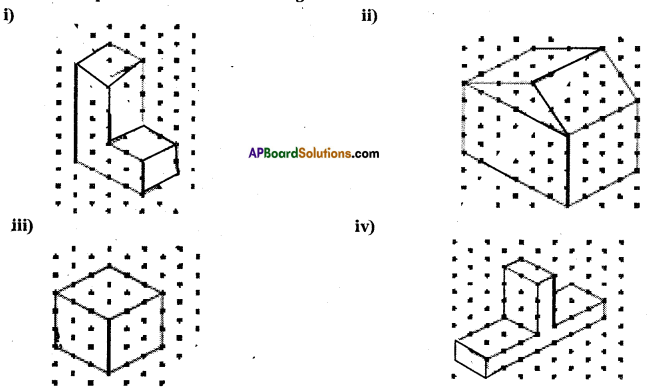Solution: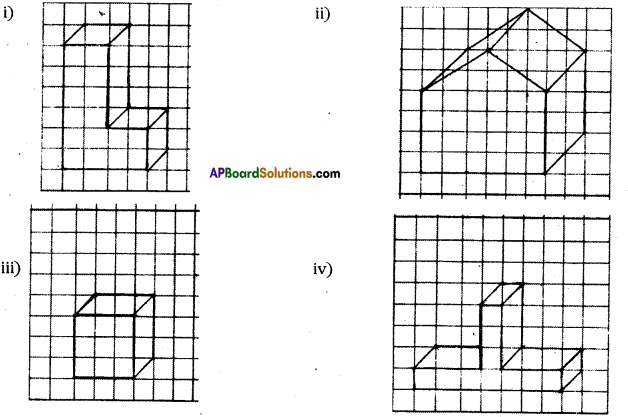Question 5.
Give (i) an oblique sketch and (ii) an isometric sketch for each of the following:
(a) A cuboid of dimensions 5 cm, 3 cm and 2 cm. (Is your sketch unique’?)
(b) A cube with an edge 4 cm long.
Solution: